# Modules¶

## Using modules¶

A python module is non other than a bunch of functions and classes, packed together. You can write your own module also, which can be used by others. Any .py file can be used as a module.

### Import¶

If Python is installed, we have the built-in modules. It is simple to import them. We did it a few times.

In :
import sys
print(sys.path)

['/home/wettl/bin/p3/lib/python36.zip', '/home/wettl/bin/p3/lib/python3.6', '/home/wettl/bin/p3/lib/python3.6/lib-dynload', '/usr/lib/python3.6', '', '/home/wettl/bin/p3/lib/python3.6/site-packages', '/home/wettl/bin/p3/lib/python3.6/site-packages/IPython/extensions', '/home/wettl/.ipython']


sys is a built-in module, it is installed by default. This module can access system files and variables (for example command line arguments).

The sys.path is a variable containing a list of strings that specifies the search path for modules.

You can import a whole modul a submodule or only one function from a module. The most frequently used commands for importing modules or a part of them:

import <modul>
import <modul> as <name>
from <modul> import <identifier>
from <modul.identifier> import <identifier>


E.g.:

In :
from csv import reader
from itertools import permutations
for p in permutations(['A', 'B', 'C']):
print(p)

('A', 'B', 'C')
('A', 'C', 'B')
('B', 'A', 'C')
('B', 'C', 'A')
('C', 'A', 'B')
('C', 'B', 'A')


### Relative import¶

You can import files from the current directory. Write the next code into a file named myfns.py:

def foo():
print("FoO")

def bar():
print("BaR")


After this we may import this by

import myfns


We can import one function only:

In :
from myfns import foo
foo()

FoO


## numpy basics¶

numpy is a module for numerical calculations. It can handle vectors, matrices, arrays and perform linear algebraic calculations, random number generation...

If you have Anaconda then it is installed by default.

You can import a module with an alternative name, just to make it shorter.

import numpy as np


The main object of numpy is ndarray, short for n-dimensional array, you can create arrays with the numpy.array function. This construct from a list of lists a 2D array, from a list of lists of lists a 3D array... The other important function is arange, which makes an array similarly to range. A range can be reshape by the reshape function:

In :
import numpy as np
x = np.arange(1, -1, -0.2)
y = np.array([[1, 2, 3], [1, 2, 4]])
z = np.arange(8).reshape(2, 2, 2)

In :
print(x)
print(y)
print(z)

[ 1.00000000e+00  8.00000000e-01  6.00000000e-01  4.00000000e-01
2.00000000e-01  2.22044605e-16 -2.00000000e-01 -4.00000000e-01
-6.00000000e-01 -8.00000000e-01]
[[1 2 3]
[1 2 4]]
[[[0 1]
[2 3]]

[[4 5]
[6 7]]]

In :
print(x.dtype, y.dtype, z.dtype)
print(x.ndim, y.ndim, z.ndim)
print(x.shape, y.shape, z.shape)

float64 int64 int64
1 2 3
(10,) (2, 3) (2, 2, 2)


You can perform elementwise operations (+ - * / **) between numpy.ndarray type objects if they are are compatible.

In :
a = np.array([20, 30, 40, 50])
b = np.arange(4)
print(a + b)
print(a - b)
print(b / (b+1.0))

[20 31 42 53]
[20 29 38 47]
[0.         0.5        0.66666667 0.75      ]


You can add a number to an array which means adding the same number to all of the elements. Same for multiplication and other operations between an array and an element.

In :
b = np.arange(10)
print(b)
print(b ** 2)
print(b + 10)
print(b % 3 == 1)

[0 1 2 3 4 5 6 7 8 9]
[ 0  1  4  9 16 25 36 49 64 81]
[10 11 12 13 14 15 16 17 18 19]
[False  True False False  True False False  True False False]


The matrix dot product is not the * operator, but the @ operation or the .dot method:

In :
A = np.arange(2, 6).reshape(2, 2)
B = np.arange(3, -1, -1).reshape(2, 2)
print(A)
print(B)

[[2 3]
[4 5]]
[[3 2]
[1 0]]

In :
print(A * B)     # elementwise
print(A @ B)     # matrix product
print(A.dot(B))  # matrix product

[[6 6]
[4 0]]
[[ 9  4]
[17  8]]
[[ 9  4]
[17  8]]


You can use the normal indexing, but there are some other comfortable possibilities:

In :
x = np.arange(15).reshape(3, 5)
print(x)
print(x[0:2])

[[ 0  1  2  3  4]
[ 5  6  7  8  9]
[10 11 12 13 14]]
[[0 1 2 3 4]
[5 6 7 8 9]]


Or you can take certain columns:

In :
print(x[2, :3])
print(x[:, 3])

[10 11 12]
[ 3  8 13]


But if you want to get a column vector (as a 2D array) use [ ]:

In :
print(x[:, ])

[[ 3]
[ 8]
]


You can use a list of indices which slices the corresponding rows (or columns).

In :
a = np.arange(12)**2
i = np.array([1, 5, 1, 3, 8])
print(a[i])

[ 1 25  1  9 64]


You can call a numpy function with an array parameter which performs (mostly) elementwise operation.

Numpy can calculate mean and standard deviation.

In :
x = np.log(np.arange(2, 10, 0.5))
print(x)
print(x.sum())
print(x.mean())
print(x.std())

[0.69314718 0.91629073 1.09861229 1.25276297 1.38629436 1.5040774
1.60943791 1.70474809 1.79175947 1.87180218 1.94591015 2.01490302
2.07944154 2.14006616 2.19722458 2.2512918 ]
26.457769829012314
1.6536106143132696
0.4600673068044455


To make an array filled with zeros or ones you can call zeros or ones (similarly to MatLab/Octave, the matrix based languages). The function eye construct identity matrix (I pronounced as eye).

In :
print(np.zeros([2, 3]))
print(np.ones([3, 1]))      # float type
print(np.eye(4, dtype=int)) # int type

[[0. 0. 0.]
[0. 0. 0.]]
[[1.]
[1.]
[1.]]
[[1 0 0 0]
[0 1 0 0]
[0 0 1 0]
[0 0 0 1]]


You can generate random numbers or array of numbers. The next function generate them with uniform distribution from $[0,1)$:

In :
np.random.rand(3, 4)

Out:
array([[0.97397551, 0.2002247 , 0.05598092, 0.78098815],
[0.61663275, 0.54883023, 0.53494673, 0.4904273 ],
[0.85622604, 0.3066138 , 0.25885798, 0.64939544]])

We get the same sequences if we set the seed:

In :
np.random.seed(2)
np.random.rand(2, 3)

Out:
array([[0.4359949 , 0.02592623, 0.54966248],
[0.43532239, 0.4203678 , 0.33033482]])

You can generate uniform numbers drawn from $[-2, 2]$ in two ways:

In :
print(np.random.rand(10)*4 - 2)
print(np.random.uniform(size=10, high=2, low=-2))

[-1.18140546  0.47708387 -0.80138131 -0.9326909   0.48453533  0.11656838
-1.46168022  0.05431249 -1.26224054  1.14134059]
[ 1.41590117 -0.02305265  1.38624594 -1.68141809  0.02098436 -1.73885398
-0.28751069 -1.61387634 -1.49136011  0.38698124]


We can generate integer sequences. E.g. let us simulate 10 dice rolls:

In :
np.random.randint(1,7,10)

Out:
array([3, 5, 3, 2, 1, 3, 6, 3, 2, 6])

## Plot¶

The matplotlib module used for plotting functions. (The %matplotlib magic function activates matplotlib interactive support. Magics are functions of the IPython distribution offering support for users of Python. jupyter uses most of them.)

In :
%matplotlib inline
import matplotlib
import matplotlib.pyplot as plt


A simple plot first. If you don't specify the $x$ values, the range $[0, 1, 2 \ldots]$ is used instead.

In :
plt.plot([1,2,4])
plt.ylabel('some numbers')
plt.show()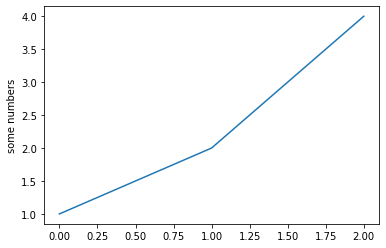These libraries cannot calculate symbolically, like Sage, just makes a series of lines between the plotted points.

Let's plot a sine curve with np.sin function. Here we generate the $x$-values with the function linespace, which divides an interval into equal parts:

In :
np.linspace(0, 1, 5)

Out:
array([0.  , 0.25, 0.5 , 0.75, 1.  ])

Draw with calculating sin at 11 points only:

In :
plt.plot(np.linspace(0, 2*np.pi, 11),
np.sin(np.linspace(0, 2*np.pi, 11)))
plt.show()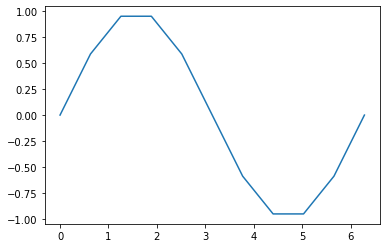In :
plt.plot(np.linspace(0, 2*np.pi, 101),
np.sin(np.linspace(0, 2*np.pi, 101)), 'g')
plt.show()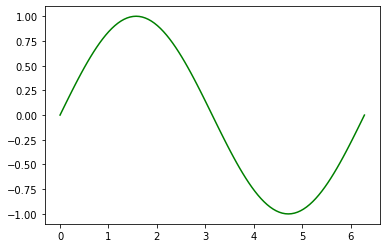In :
plt.plot(np.linspace(0, 2*np.pi, 101),
np.sin(np.linspace(0, 2*np.pi, 101)), 'k')
plt.axis([0, 2*np.pi, -2, 2])
plt.show()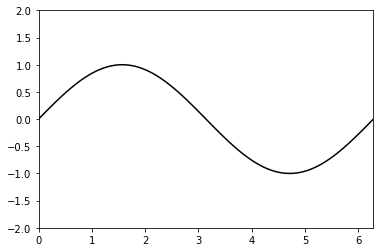In :
plt.plot(np.linspace(0, 2*np.pi, 101),
np.sin(np.linspace(0, 2*np.pi, 101)), 'r')
plt.axis('equal')
plt.show()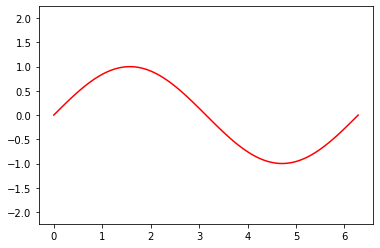### Monte–Carlo-method¶

Monte–Carlo simulation is an easy but not too fast way to estimate an integral.

To calculate $\int_{-2}^2e^{-x^2}\,\mathrm{d}x$ you draw random points in the rectangle $[-2,2]\times[0,1]$ and count how many points fell under the graph: $e^{-x^2} > y$. In the array J we store the indexes of these $(x,y)$ points.

The ratio of the points under the curve times the size of the rectangle is an approximation of the area.

First let us study the function where:

In :
a = np.arange(4).reshape(2, 2)
print(a)
print((np.where(a % 2)))
b = np.arange(4)
print(b)
print((np.where(b % 2)))

[[0 1]
[2 3]]
(array([0, 1]), array([1, 1]))
[0 1 2 3]
(array([1, 3]),)

In :
n = 500000
X = np.random.rand(n, 2)
X[:, 0] = X[:, 0]*4 - 2
J = np.where(X[:, 1] < np.exp(-X[:, 0]**2))
print(4 * len(J) / n)

1.75776


In a picture:

In :
Xp = X[:2000]
Ip = [i for i in range(2000) if i in J]
Inp = [i for i in range(2000) if i not in J]
plt.plot(Xp[Ip, 0], Xp[Ip, 1], 'bd', Xp[Inp, 0], Xp[Inp, 1], 'r.')
plt.show()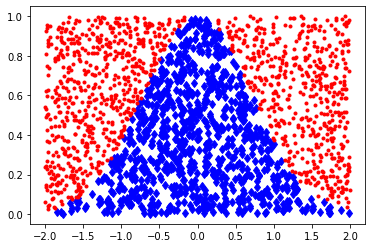In [ ]: